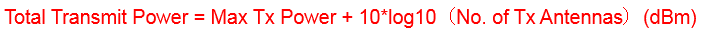# 5G NR Total Transmit Power | Maximum Cell Transmit Power | Reference Signal Power

The Reference Signal Power and the total transmit power of the cell can calculated by using a single channel power using following formula,• Maximum Transmit Power indicates the transmit power in dBm per single channel
• Reference Signal Power is the power in dBm per RE of single channel
• RBcell indicate total RB number based on the cell bandwidth each RB with 12REs

Example Calculation

Consider a system is configured with Max Transmit Power as 40 dBm (10 watt per channel) and calculation can be done with different sub-carrier spacing

Sub-carrier Spacing 15 KHz 270 RBs with 50MHz

• Reference Signal Power = 40 – 10 x log10（270 x 12)  = 40 – 35.10
• Reference Signal Power = 4.9 dBm

Sub-carrier Spacing 30 KHz 273 RBs with 100MHz

• Reference Signal Power = 40 – 10 x log10（273 x 12)  = 40 – 35.15
• Reference Signal Power = 4.85 dBm

Sub-carrier Spacing 60 KHz 130 RBs with 100MHz

• Reference Signal Power = 40 – 10 x log10（130 x 12)  = 40 – 31.93
• Reference Signal Power = 8.07 dBm

If you not able to co-related the carrier spacing with RB count please read our older post 5G New Radio Throughput Calculation embedded PDF page 6 or NR Resource Block Definition and RBs Calculation

The total transmit power of NR base station can be calculated taking Max Transmit power and No. of Tx antenna into account with following formula.Consider same 40 Bm as cell Max power, the total Tx power can be calculated for different antenna configurations e.g. 8 antenna , 16 antenna, 64 antenna and 128 antenna systems.

• Total Transmit Power with 8 Tx Antenna = 40 + 10 x log10 (8) = 40 + 9.03            =49.03 dBm
• Total Transmit Power with 16 Tx Antenna = 40 + 10 x log10 (16) = 40 + 12.04       =52.04 dBm
• Total Transmit Power with 64 Tx Antenna = 40 + 10 x log10 (64) = 40 + 18.06      =58.06 dBm
• Total Transmit Power with 128 Tx Antenna = 40 + 10 x log10 (128) = 40 + 21.07   =61.07 dBm

Note: Total Transmit Power had included antenna gain (directive gain in dBi) which is used to calculated Equivalent Isotropical Radiated Power (EIRP).

Related Post: